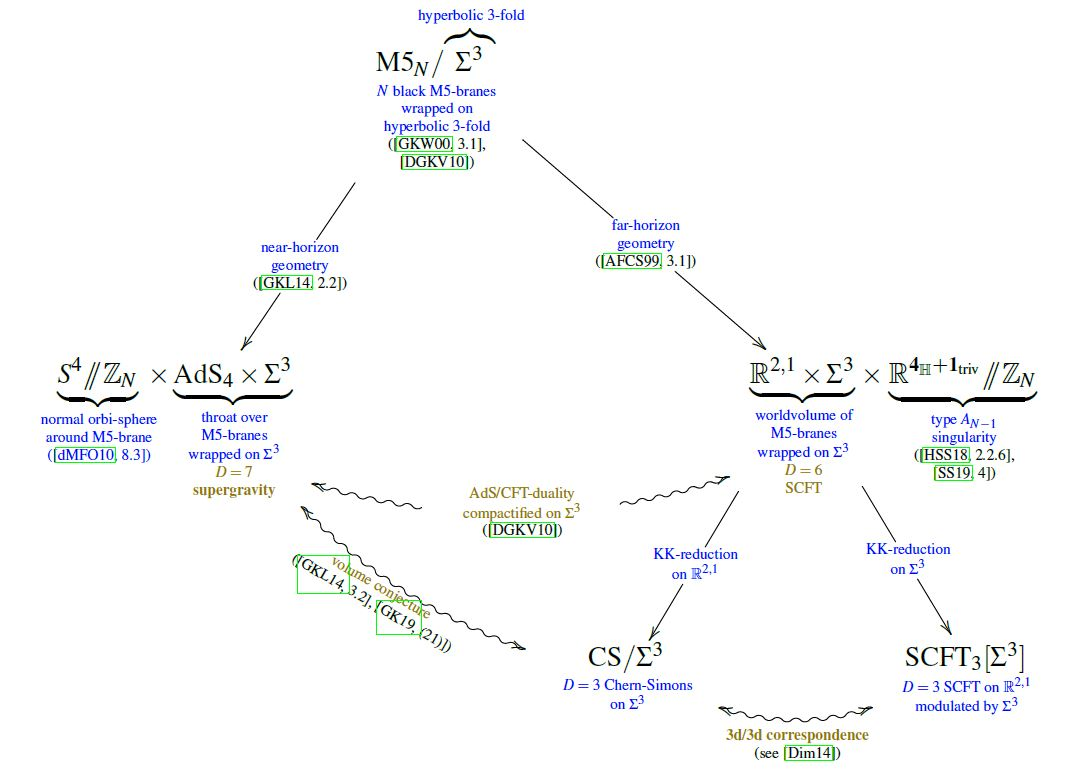# nLab 3d-3d correspondence

Contents

### Context

#### Duality in string theory

duality in string theory

general mechanisms

string-fivebrane duality

string-string dualities

M-theory

F-theory

string-QFT duality

QFT-QFT duality:

# Contents

## Properties

### Relation to volume conjecture

In Gang-Kim-Lee 14b, 3.2, Gang-Kim 18 (21) it is argued that the volume conjecture for Chern-Simons theory on hyperbolic 3-manifolds $\Sigma^3$ is the combined statement of two dualities in string theory

for the situation of M5-branes wrapped on $\Sigma^3$ (DGKV 10):graphics from Sati-Schreiber 19c

Wrapping the M5-brane on a torsion 3-cycle yields: fractional M2-brane.

Wrapping the M5-brane instead on a 2-manifold yields: AGT correspondence.

### General

Original articles include

Specifically for Seifert 3-manifolds (such as lens spaces):

Review is in

Relation of the AGT-correspondence to the D=6 N=(2,0) SCFT and the 3d-3d correspondence:

Discussion of D=11 N=1 supergravity solutions for the near horizon geometry of black M5-branes wrapped on hyperbolic 3-manifolds $\Sigma^3 = H^3/\Gamma$:

### Relation to perturbative CS-observables

specifically to Reidemeister torsion:

### Relation to volume conjecture

Discussion of the volume conjecture by combining the 3d/3d correspondence with AdS/CFT in these backgrounds:

Enhanced to a defect field theory:

More in:

• Jin-Beom Bae, Dongmin Gang, Jaehoon Lee, 3d $\mathcal{N}=2$ minimal SCFTs from Wrapped M5-branes, JHEP 08 (2017) 118 (arXiv:1610.09259)

### Knot invariants

For discussion of knot invariants:

### Entropy computation

Applied to computation of Bekenstein-Hawking entropy for black holes in string theory: